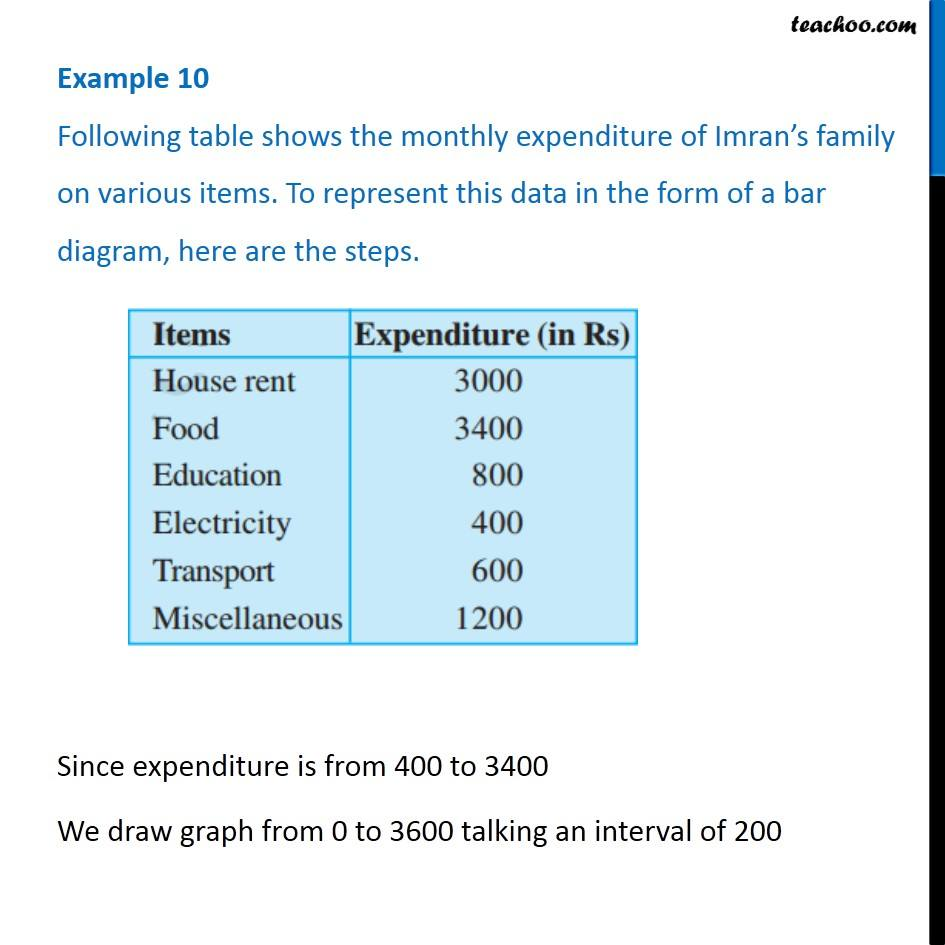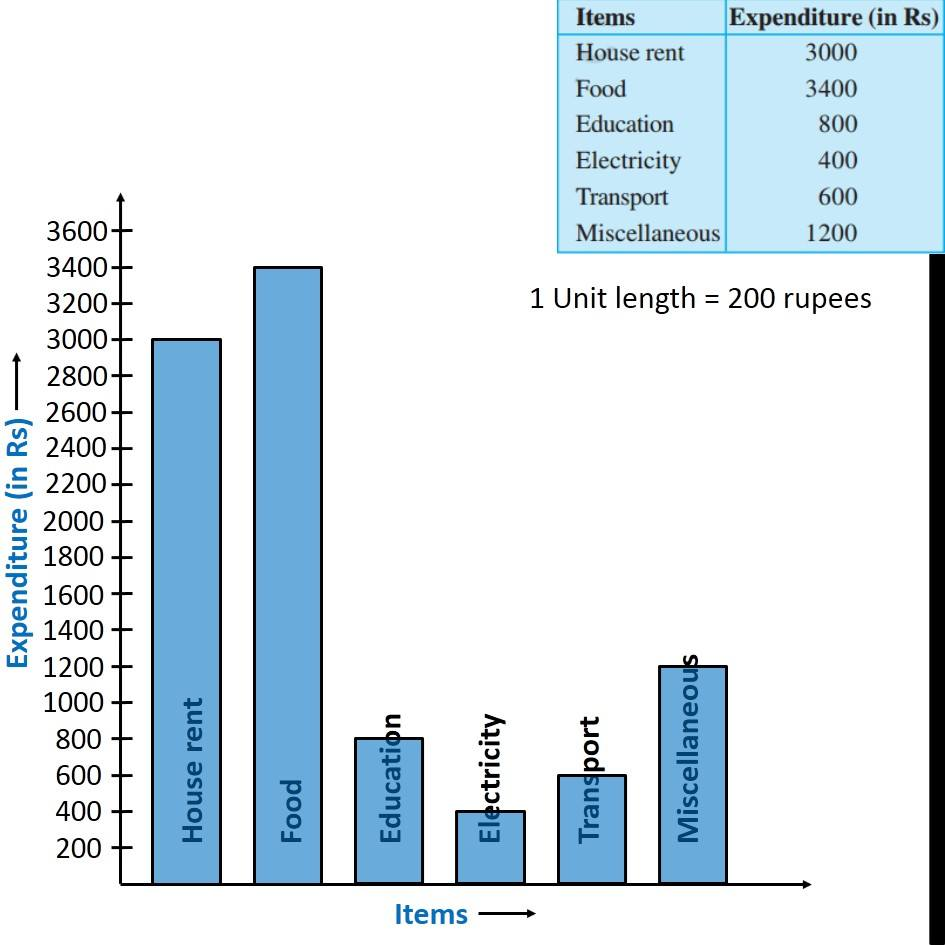1. Chapter 9 Class 6 Data Handling
2. Serial order wise
3. Examples

Transcript

Example 10 Following table shows the monthly expenditure of Imran’s family on various items. To represent this data in the form of a bar diagram, here are the steps. Since expenditure is from 400 to 3400 We draw graph from 0 to 3600 talking an interval of 200 1 Unit length = 200 rupees

Examples